# Decimals - Adding and Subtracting

To add and subtract decimal numbers we can use the standard methods as shown in Written methods of Addition and Written methods of Subtraction. We just have to make sure that the decimal point is included and to be very careful to line up the numbers in their correct place value columns. The decimal points must line up.

### Lets try an addition with decimal numbers: say,  4.23 + 3.14

As usual, we begin with the numbers in the column on the far right, in this case the 1/100 column.

3 + 4 = 7, put the 7 at the bottom of the column.

Next, we move on to the 1/10 column,

2 + 1 = 3, put the 3 at the bottom of the column.

Next, we move on to the units column,

4 + 3 = 7, put the 7 at the bottom of the column.

The calculation is now complete,

### 4.23 + 3.14 = 7.37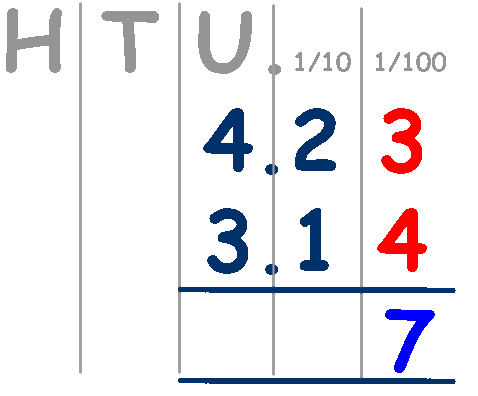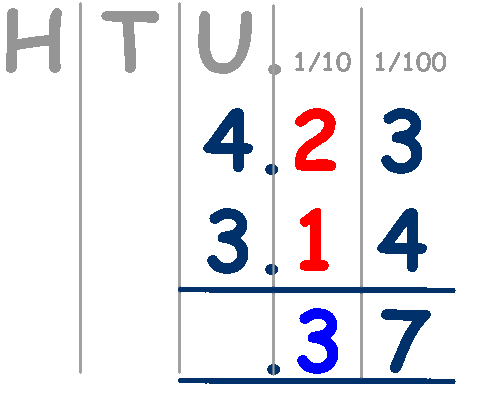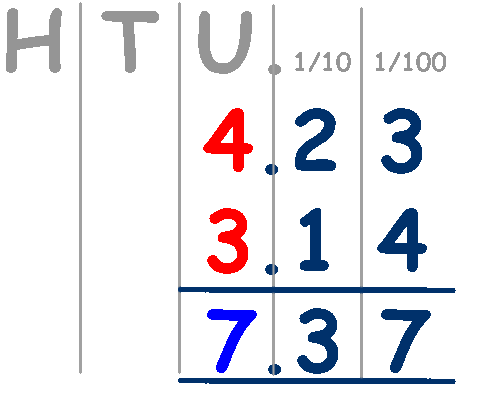### Lets try a subtraction with decimal numbers: say, 25.65 - 12.23

As usual, we begin with the numbers in the column on the far right, in this case the 1/100 column.

5 - 3 = 2, put the 2 at the bottom of the column.

Next, we move on to the 1/10 column,

6 - 2 = 4, put the 4 at the bottom of the column.

Next, we move on to the units column,

5 - 2 = 3, put the 3 at the bottom of the column.

Next, we move on to the tens column,

2 -1 = 1, put the 1 at the bottom of the column.

The calculation is now complete,

### 25.65 - 12.23 = 13.42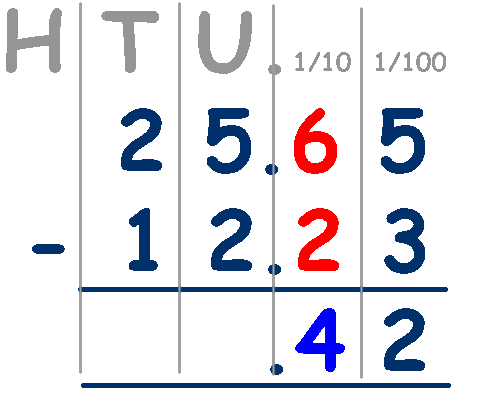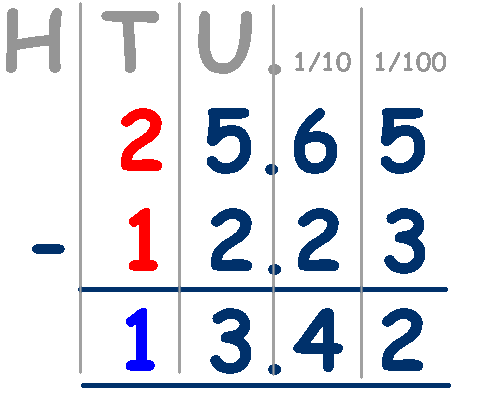## Remember the zero's

If the number includes zeros, don't forget to include them. You may see a number like, 0.05, ensure that the decimal points are lined up and include the zero's.

If the numbers have different lengths, like 24.2 and 31.76, adjust the length of the numbers so that they are the same length by adding zero's to the shorter number,
24.2 becomes 24.20 - it still has the same value.

Try these worksheets from Maths is Fun

and these word problems from Primary Resources